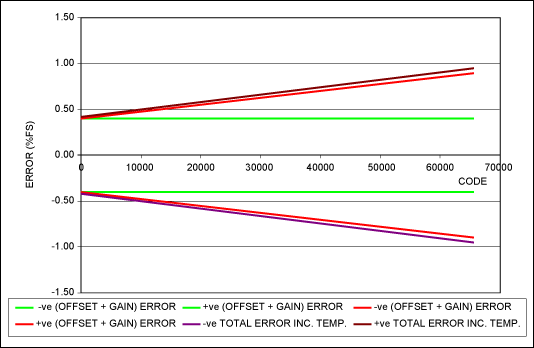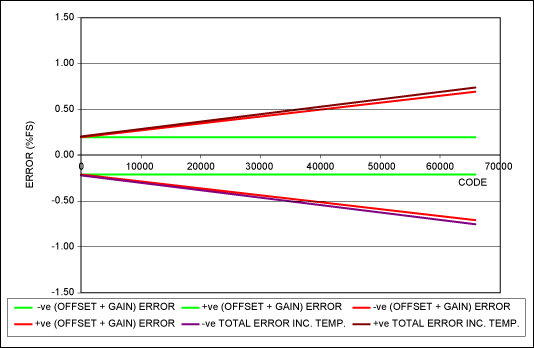# Understanding Temperature Drift in a Precision Digital-to-Analog Converter (DAC)

### Abstract

This application note analyzes the external influences that introduce error to a precision digital-to-analog converter (DAC). Focus will be on temperature drift. This error is recognized as part of a DAC's error budget. The article discusses the error factors introduced by both the data converter and the voltage reference. After understanding the sources of error, it then provides the calculations required to specify the data converter and that will compensate for the error and meet a system's target specifications.

This application note focuses on Maxim's 3-terminal voltage references and precision DACs. Voltage references and DACs have many specifications, but only those relevant to the error budget will be discussed.

### Overview

An ideal digital-to-analog converter (DAC) produces an analog output voltage or current that is perfectly linear and independent of many external influences such as temperature. That said, DACs are, of course, subject to errors caused by many external factors, notably temperature. As temperature varies, the DAC can drift. This is of particular importance when a precision DAC is used to set a precise bias value. Any error can be calibrated out at room temperature. However, variations with temperature are far more difficult to compensate for. The errors that drift most with temperature are dominated by offset error and gain error.
This application note describes how DAC offset and gain errors are specified with temperature. It shows how a designer can anticipate the errors in the design process. Once understood, this knowledge can be used to ensure that a system meets its required specification over temperature.

### Offset and Gain Errors

As stated above, DAC performance is affected by many sources of error including offset error and gain error. These factors are specified in the "Static Accuracy" section of a DAC's data sheet. An example is shown in Figure 1 for the MAX5134 16-bit, quad DAC.Note 4: Gain and offset tested within 100mV of GND and AVDD.
Figure 1. The MAX5134 offset and gain errors.
What do these specifications really mean for DAC performance?
Offset error defines how well the actual transfer function of a DAC matches the ideal at a single point. For a unipolar output, this is at code zero. This error is often called zero-code error. For a bipolar output, this is at the point where the DAC output should pass through zero.
Gain error is a measure of the slope of the transfer function. In the example device, the slope can be between 99.5% and 100.5% of ideal.
Ideal offset and gain errors are shown in Figure 2. Note that offset and gain errors can be both positive and negative.Figure 2. Offset and gain errors.
Offset and gain errors are not generally measured directly. If a unipolar device exhibits a negative offset error, then measuring at code zero will give an erroneous result. The explanation for this is actually straightforward. Theoretically, with a negative offset error the output should be negative at code zero. A unipolar DAC cannot do that, since it generally only has a positive supply. Thus, two points are measured and the offset and gain errors are calculated. One point is close to code zero while the other is close to, or possibly at, maximum code. The MAX5134, for example, is tested within 100mV of ground and AVDD; the analog supply voltage as described in Note 4 of Figure 1.
Now consider the influence of temperature. Both offset and gain errors drift with temperature. This is of particular importance where a DAC is used to set precise bias values. Fixed-offset and gain error can be calibrated out using various techniques. (See application note 4494, "Methods for Calibrating Gain Error in Data-Converter Systems," for some ideas on this.) However, calibrating out temperature drift is far more complex since temperature must first be measured and a variable compensation applied dependant on temperature.

### Example Calculations and Typical Results

Using the MAX5134 as an example, we can calculate the maximum static errors that we will see over large numbers of devices. First, we need to define some equations that will enable us to calculate the extent of the errors.
VOUT = N × G × (GE + GET) + OE + OETWhere: VOUT = the output voltage N = DAC code G = DAC gain GE = DAC gain error GET = additional gain error from temperature effects OE = DAC offset error OET = additional offset error from temperature effects VREF = the reference voltage NMAX = the maximum DAC code
The offset-error drift is specified as ±4µV/°C. This is specified using the box method. (See application note 4300, "Calculating the Error Budget in Precision Digital-to-Analog Converter (DAC) Applications," for a further description.) To determine the offset over temperature, we multiply the drift by the specified temperature range. Note that this is the specified operating range for the part, not the operating range of the application. In this case, that range is -40°C to +105°C. Therefore, the offset drift over temperature is ±0.58mV. Similarly, the gain temperature coefficient is specified as 2ppm/°C, which equates to ±0.029%FS (full-scale) total.
We use VREF = 2.5V as the first example. In this case, we have a 16-bit DAC so NMAX = 65535.
Now we have a slight problem. The offset and gain errors are specified as "min/max" values, which is helpful. However, the temperature effects are only specified as typical (typ) values. We could use these typical values or estimate by experience how they would vary across all lots. For the moment we just use typ values.
If we plot the output voltage including the initial error vs. code, we get the plot shown in Figure 3. Since this is a plot of a real DAC, the lines are much closer together than in Figure 2. Therefore, it is better to plot the deviation from ideal. This is shown in Figure 4. Also shown in Figure 4 is the total error, including temperature effects.Figure 3. Example DAC output vs. code, showing the extents of the gain and offset errors, VREF = 2.5V.Figure 4. Example DAC output error vs. DAC code, VREF = 2.5V.
We see immediately that the temperature effects are very much smaller than the initial error. Therefore, even though the data sheet specifies typ values only for the temperature effects, the total error will not be significantly compromised by this. The total error is ±0.423%FS (±10.6mV) at code zero and ±0.952%FS (±23.8mV) at maximum code.
There may be some improvements to be made. If the reference voltage is increased, gain errors will increase in absolute terms since they are specified as %FS. However, offset errors will stay the same in absolute terms. The effect of increasing the reference voltage is, thus, to increase the full-scale voltage. We could then divide down the DAC output externally to the required voltage. This would effectively divide the gain error back to its original value. However, offset error would also be divided. Figure 5 shows the effect of such a scheme.Figure 5. Example DAC output error vs. DAC code, VREF = 2.5V.
The total error is ±0.212%FS (±5.3mV) at code zero and ±0.740%FS (±18.5mV) at maximum code.
We have, of course, ignored any error involved in the output divider. However, this approach is reasonable since precision voltage-dividers can be used. The MAX5490 voltage-divider can, for example, achieve ±0.05% ratio accuracy over temperature. Of course the disadvantage of dividing the DAC's output is that we lose the drive capability. This can be restored using an amplifier, but this would add error itself. Discussions of this tactic are beyond the scope of this applications note.

### Conclusions

We defined offset and gain errors that affect DACs. We showed by example how to calculate the worst-case errors that would be present and gave a typical example. We also suggested a possible method to improve the total error.